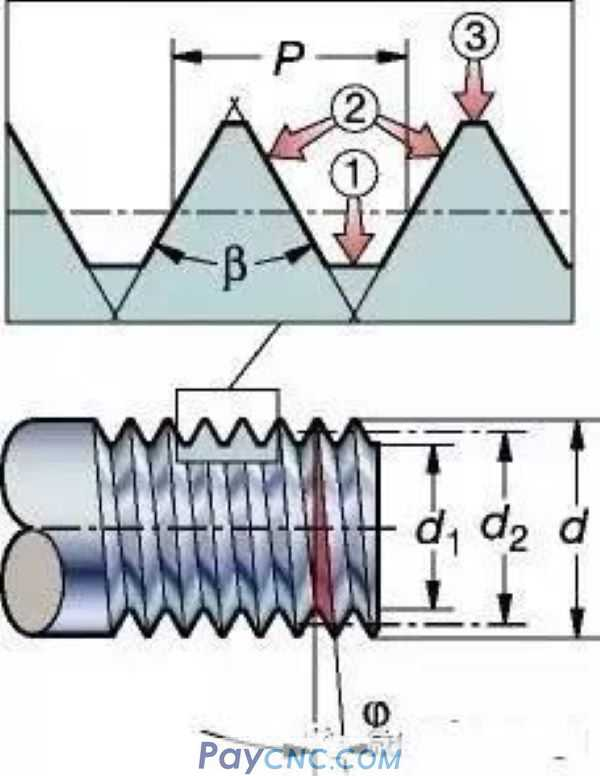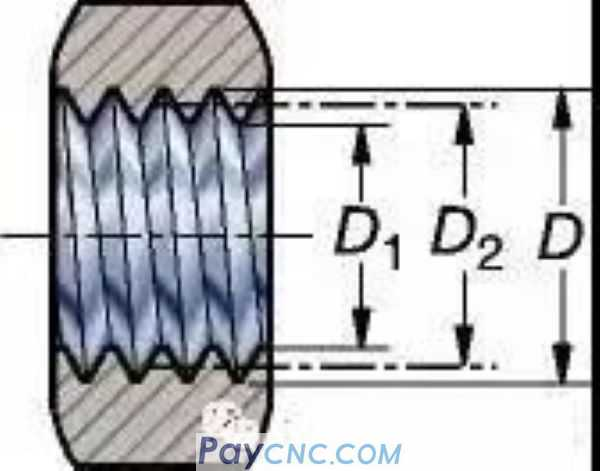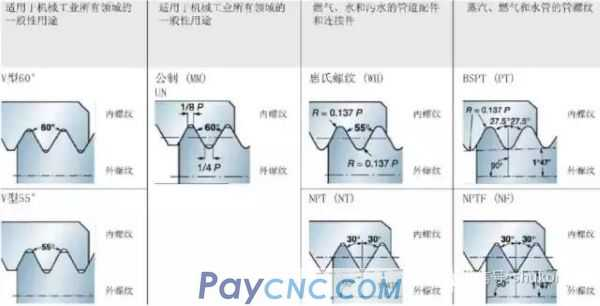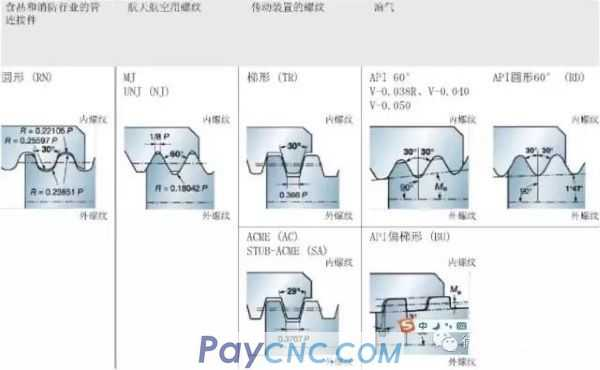1. What is a thread?   A thread is a helix cut into the workpiece from the outside or the inside. The main functions of the thread are: 1. Form a mechanical connection by combining the internal threaded product and the external threaded product. 2. Transfer motion by converting rotary motion to linear motion, and vice versa. 3. Obtain mechanical advantages.   2. Thread profile and terminology The thread profile determines the geometry of the thread, including the workpiece diameter (major diameter, middle diameter and minor diameter); thread profile angle; thread pitch and helix angle.         1. Thread terminology① Tooth bottom: the bottom surface connecting two adjacent thread flanks. ②Flanks: the side surface of the thread connecting the top and bottom of the tooth. ③Crest: the top surface connecting the two flanks. P = pitch, mm or number of threads per inch (t.p.i.) ß = tooth angle ϕ = thread helix angle d = Major diameter of external thread D = Major diameter of internal thread d1 = minor diameter of external thread D1 = Internal thread minor diameter d2 = pitch diameter of external thread D2 = Internal thread pitch diameterPitch diameter, d2 / D2   The effective diameter of the thread. Approximately halfway between the major and minor paths.The geometry of the thread is based on the thread pitch (d, D) and pitch (P): the axial distance along the thread from one point on the thread to the corresponding next point on the workpiece. This can also be seen as a triangle detoured from the workpiece.vc = cutting speed (m/min) ap = total thread depth (mm) nap = total thread depth (mm) t.p.i. = number of threads per inch Feed = pitch   2. Ordinary thread profile1. Calculation and tolerance of external thread pitch diameter of 60° tooth profile (GB197/196) a. Calculation of the basic size of the pitch diameter   The basic size of the pitch diameter of the thread = the major diameter of the thread-the pitch × the coefficient value. Formula: d/D-P×0.6495 Example: Calculation of the pitch diameter of external thread M8 8-1.25×0.6495=8-0.8119≈7.188 b. Commonly used 6h external thread pitch tolerance (based on the pitch) The upper limit value is "0" The lower limit is P0.8-0.095, P1.00-0.112, P1.25-0.118 P1.5-0.132P1.75-0.150P2.0-0.16 P2.5-0.17 The upper limit calculation formula is the basic size, and the lower limit calculation formula d2-hes-Td2 is the basic size-deviation-tolerance of the pitch diameter. M8's 6h-level pitch diameter tolerance value: the upper limit is 7.188 and the lower limit is 7.188-0.118=7.07. C. The basic deviation of the commonly used 6g level external thread pitch diameter: (based on the pitch) P0.80-0.024P1.00-0.026P1.25-0.028P1.5-0.032 P1.75-0.034P2-0.038P2.5-0.042 The upper limit calculation formula d2-ges is the basic size-deviation The lower limit calculation formula d2-ges-Td2 is the basic size-deviation-tolerance Example M8's 6g-level pitch diameter tolerance value: upper limit 7.188-0.028=7.16 lower limit: 7.188-0.028-0.118=7.042. Note: ①The above thread tolerances are based on coarse threads, and there are some changes to fine thread tolerances, but they are only larger tolerances, so the control will not exceed the specification limits according to this control, so they are not marked in the above. Out. ②The diameter of the threaded polished rod blank is increased by 0.04-0.08 compared to the design thread pitch diameter in actual production according to the accuracy required by the design and the extrusion force of the thread processing equipment. It is the diameter of the threaded polished rod blank. Our company's M8 external thread 6g level threaded polished rod blank diameter is actually in the range of 7.08-7.13. ③Considering the needs of the production process, the lower control limit of the pitch diameter of the external thread in the actual production without heat treatment and surface treatment should be kept at 6h as far as possible.   2. Calculation and tolerance of 60° internal thread pitch diameter (GB197/196) a.6H-level thread pitch tolerance (based on the pitch) Upper limit: P0.8+0.125P1.00+0.150P1.25+0.16P1.5+0.180 P1.25+0.00P2.0+0.212P2.5+0.224 The lower limit is "0", The upper limit calculation formula 2+TD2 is the basic size + tolerance. Example M8-6H internal thread pitch diameter is: 7.188+0.160=7.348 upper limit: 7.188 is the lower limit. b. The calculation formula of the basic size of the inner thread's pitch diameter is the same as that of the outer thread That is, D2=D-P×0.6495, that is, the pitch diameter of the internal thread is equal to the major diameter of the thread-the pitch × the coefficient value. c. The basic deviation of 6G thread pitch diameter E1 (based on the pitch)   P0.8+0.024P1.00+0.026P1.25+0.028P1.5+0.032 P1.75+0.034P1.00+0.026P2.5+0.042   Example: the upper limit of the pitch diameter of M86G internal thread: 7.188+0.026+0.16=7.374 Lower limit: 7.188+0.026=7.214   The upper limit formula 2+GE1+TD2 is the basic size of the pitch diameter + deviation + tolerance The lower limit formula 2+GE1 is the pitch diameter + deviation   Third, the calculation and tolerance of the major diameter of the external thread (GB197/196) a. 6h upper limit of major diameter of external thread That is, the thread diameter value example M8 is φ8.00 and the upper limit tolerance is "0". b. 6h-class major diameter lower limit tolerance of external thread (based on pitch) P0.8-0.15P1.00-0.18P1.25-0.212P1.5-0.236P1.75-0.265 P2.0-0.28P2.5-0.335   The formula for calculating the lower limit of the major diameter: d-Td is the basic size of the major diameter of the thread-tolerance. Example: M8 external thread 6h major diameter size: the upper limit is φ8, the lower limit is φ8-0.212=φ7.788 c. Calculation and tolerance of 6g major diameter of external thread Benchmark deviation of 6g external thread (based on pitch) P0.8-0.024P1.00-0.026P1.25-0.028P1.5-0.032P1.25-0.024P1.75--0.034 P2.0-0.038P2.5-0.042   The upper limit calculation formula d-ges is the basic size of the major diameter of the thread-the reference deviation The lower limit calculation formula d-ges－Td is the basic size of the major diameter of the thread-reference deviation-tolerance Example: The upper limit of the 6g major diameter of M8 external thread is φ8-0.028=φ7.972. Lower limit φ8-0.028-0.212=φ7.76   Note: ① The major diameter of the thread is determined by the diameter of the polished rod blank and the degree of wear of the thread rolling plate/rolling wheel, and its value appears inversely proportional to the pitch diameter of the thread on the basis of the same blank and thread processing tools. That is, the smaller the diameter is, the larger the diameter, and the larger the diameter, the smaller the larger diameter. ②For parts that need to be processed by heat treatment and surface treatment, considering the relationship of the processing process, the major diameter of the thread should be controlled at the lower limit of 6h plus 0.04mm or more in actual production, such as the external thread of M8 is rolling (rolling) The major diameter of the wire should be ensured to be above φ7.83 and below 7.95.   Fourth, the calculation and tolerance of the small diameter of the internal thread a. Calculation of the basic size of the small diameter of the internal thread (D1) Basic size of thread minor diameter = basic size of internal thread-pitch × coefficient Example: The basic size of the minor diameter of the internal thread M8 is 8-1.25×1.0825=6.646875≈6.647 b. The small diameter tolerance of internal thread 6H (based on the pitch) and the calculation of the small diameter value P0.8+0.2P1.0+0.236P1.25+0.265P1.5+0.3P1.75+0.335 P2.0+0.375P2.5+0.48   The lower limit deviation formula D1+HE1 of internal thread 6H grade is the basic size of internal thread minor diameter + deviation. Note: The lower bias value of 6H level is "0"   The calculation formula for the upper limit value of internal thread 6H level = D1 + HE1 + TD1 is the basic size of the small diameter of the internal thread + deviation + tolerance. Example: The upper limit of the minor diameter of the 6H M8 internal thread is 6.647+0=6.647 The lower limit of the minor diameter of the 6H M8 internal thread is 6.647+0+0.265=6.912 c. The basic deviation of the minor diameter of internal thread 6G (based on the pitch) and the calculation of the minor diameter value P0.8+0.024P1.0+0.026P1.25+0.028P1.5+0.032P1.75+0.034 P2.0+0.038P2.5+0.042   The formula for the lower limit of the minor diameter of the internal thread 6G level = D1 + GE1 is the basic size of the internal thread + deviation. Example: The lower limit of the minor diameter of the 6G M8 internal thread is 6.647+0.028=6.675 The upper limit formula D1+GE1+TD1 of the minor diameter of the 6G M8 internal thread is the basic size of the internal thread + deviation + tolerance. Example: The upper limit of the minor diameter of 6G M8 internal thread is 6.647+0.028+0.265=6.94 Note: ①The tooth height of the internal thread is directly related to the load moment of the internal thread, so it should be within its 6H upper limit in the production of blanks. ②In the process of internal thread processing, the smaller the diameter of the internal thread will affect the use efficiency of the tool-the use of the tap. From the perspective of use, the smaller the diameter, the better, but in general considerations, the smaller diameter is generally used in the middle Limit to the upper limit, if it is cast iron or aluminum, it should be between the lower limit to the middle limit of the minor diameter. ③The small diameter of internal thread 6G can be implemented as 6H in the production of blanks. Its accuracy grade mainly considers the plating of the pitch diameter of the thread. Therefore, only the pitch diameter of the tap is considered during thread processing, and the minor diameter of the light hole is not considered.   5. Calculation formula of indexing head single indexing method Calculation formula of single division method: n=40/Z n: the number of revolutions that the indexing head should turn Z: equal fraction of artifacts 40: fixed number of indexing head Example: Calculation of milling six squares Substituting into the formula: n=40/6 Calculation: ① Simplify the fraction: find the smallest divisor 2 to reduce, that is, divide the numerator and denominator by 2 at the same time to get 20/3. While the fraction is reduced, its equal division remains unchanged. ②Calculate the fraction: At this time, it depends on the numerical value of the numerator and denominator; if the numerator and the denominator are large, calculate it. 20÷3=6(2/3) is the value of n, that is, the indexing head should be rotated by 6(2/3). At this time the score has become a mixed number; the integer part 6 of the mixed number is the index The head should have turned 6 full turns. The score 2/3 with a score can only be 2/3 of a turn, and it must be recalculated at this time. ③Selection and calculation of the indexing plate: The calculation of less than one circle must be realized with the indexing plate of the indexing head. The first step in the calculation is to expand the score 2/3 at the same time. For example: If the score is expanded by 14 times at the same time 28/42; If you expand by 10 times at the same time, the score will be 20/30; if you expand by 13 times at the same time, the score will be 26/39... The number of expansion multiples depends on the number of holes on the indexing plate. At this time, you should pay attention to:   ①The number of holes in the indexing plate must be divisible by the denominator 3. For example, 42 holes in the previous example are 14 times of 3, 30 holes are 10 times of 3, and 39 is 13 times of 3... Click here to learn macro program , Only 9.9 yuan per lesson ②The expansion of the score must be the expansion of the numerator and denominator at the same time, and its equal division remains unchanged, as in the example 28/42=2/3×14=(2×14)/(3×14); 20/30=2/3×10=(2×10)/(3×10); 26/39=2/3×13=(2×13)/(3×13)   The denominator 42 of 28/42 means that the 42 holes of the number of divisions are used for indexing; the numerator 28 means that the numerator 28 is forwarded on the positioning hole of the upper wheel and then turned forward through 28 holes or 29 holes on the positioning hole of the current wheel, 20/30 is at 30 The index plate of holes is rotated forward by 10 holes, that is, 11 holes are the positioning holes of the current wheel. 26/39 is the indexing plate of 39 holes is rotated forward and 26 holes, or 27 holes, are the positioning holes of the current wheel. When milling a hexagon (six equal parts), you can use 42 holes, 30 holes, 39 holes and other holes divisible by 3 as the index: the operation is to turn the handle 6 times, and then move forward on the positioning holes of the upper wheel. Then turn 28+1/10+1/26+! The 29/11/27 hole of the hole is used as the positioning hole of this wheel. Example 2: Calculation for milling a 15-tooth gear. Substituting into the formula: n=40/15 Calculate n=2(2/3) It is to turn 2 full circles and then select the indexing holes that are divisible by 3, such as 24, 30, 39, 42.51.54.57, 66, etc. The orifice plate and then turn forward 16, 20, 26, 28, 34, 36, 38, 44 Add 1 hole, namely 17, 21, 27, 29, 35, 37, 39, 45 as the positioning hole of this wheel. Example 3: Index calculation for milling 82 teeth. Substitute into the formula: n=40/82 Calculate n=20/41 That is, as long as you choose the indexing plate with 41 holes, turn 20+1 or 21 holes on the positioning hole of the upper wheel as the positioning hole of the current wheel. Example 4: Index calculation for milling 51 teeth   Substitute the formula n=40/51. Because the score cannot be calculated at this time, you can only choose the hole directly, that is, choose the 51-hole indexing plate, and then turn 51+1 on the upper wheel positioning hole, that is, 52 holes as the positioning hole of this wheel. That is. Example 5: Index calculation for milling 100 teeth. Substitute into the formula n=40/100 Calculate n=4/10=12/30 That is, select a 30-hole indexing plate, and then turn 12+1 or 13 holes on the positioning hole of the upper wheel as the positioning hole of the current wheel. If all the index plates do not have the required number of holes for calculation, the double index method should be used for calculation, which is not included in this calculation method. In actual production, gear hobbing is generally used, because the actual operation after the double index calculation is extremely inconvenient. 6. The calculation formula of a circle inscribed with a hexagon ①Find the opposite side of circle D (S surface) S=0.866D is the diameter × 0.866 (coefficient) ②Find the diameter of circle (D) on opposite sides of the hexagon (S surface) D=1.1547S i.e. opposite side×1.1547 (coefficient)   Seven, the calculation formula of the six sides and diagonals of the cold heading process ①The opposite side of the outer hexagon (S) find the opposite angle e e=1.13s is the opposite side×1.13 ②The opposite side of the inner hexagon (s) to find the opposite angle (e) e=1.14s, the opposite side×1.14 (coefficient) ③The opposite side of the outer hexagon (s) to find the diameter of the head of the opposite angle (D) The diameter of the circle (D) should be calculated according to (the second formula in the sixth) on the opposite side of the hexagon (s surface) and the offset center value should be increased appropriately, that is, D≥1.1547s. The offset center amount can only be estimated.   8. The calculation formula of a square inscribed by a circle ①The circle (D) finds the opposite side of the square (S surface) S=0.7071D is the diameter×0.7071 ②The opposite side of the square (S surface) to find a circle (D) D=1.414S is the opposite side×1.414   Nine, the calculation formula of the square opposite and diagonal of the cold heading process ①The opposite side of the outer square (S) find the opposite angle (e) e=1.4s is the opposite side (s)×1.4 parameter ②The opposite side of the inner square (s) find the opposite angle (e) e=1.45s is the opposite side (s)×1.45 series  number   10. The calculation formula of the volume of the hexagonal cube s20.866×H/m/k means opposite side×opposite side×0.866×height or thickness.   11. Volume calculation formula of truncated cone (cone) body   0.262H (D2+d2+D×d) means 0.262×height×(big head diameter×big head diameter+small head diameter×small head diameter+big head diameter×small head diameter).   12. Volume calculation formula of spherical missing body (such as semi-circular head)   3.1416h2 (R-h/3) is 3.1416 × height × height × (radius-height ÷ 3).   13. Calculation formula of processing size of taps for internal thread 1. Calculation of the major diameter D0 of the tap D0=D＋(0.866025P/8)×(0.5~1.3) That is, the basic size of the large diameter thread of the tap + 0.866025 pitch ÷ 8×0.5 to 1.3. Note: How much to choose from 0.5 to 1.3 should be confirmed according to the size of the pitch, the larger the pitch value, the smaller the coefficient should be used, on the contrary, the smaller the pitch value, the larger the coefficient should be used accordingly. 2. Calculation of the pitch diameter of the tap (D2)   D2=(3×0.866025P)/8 that is, the pitch diameter of the tap=3×0.866025×the pitch ÷ 8 3. Calculation of tap diameter (D1)   D1=(5×0.866025P)/8 i.e. tap diameter=5×0.866025×pitch ÷ 8   14. The calculation formula for the length of various shapes of cold heading materials   Known: The volume formula of a circle is diameter×diameter×0.7854×length or radius×radius×3.1416×length. Namely d2×0.7854×L or R2×3.1416×L When calculating, the volume X÷diameter÷diameter÷0.7854 or X÷radius÷radius÷3.1416 is the length of the material to be used. Column formula = X/(3.1416R2) or X/0.7854d2 The X in the formula represents the volume value of the required material; L represents the actual length of the material; R/d represents the radius or diameter of the actual feeding material.